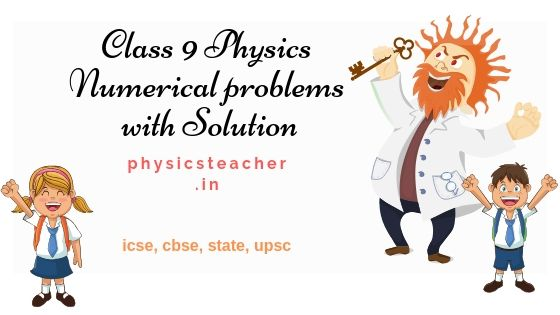# Physics Numericals Class 9 Motion & other Chapters solved

Last updated on December 4th, 2023 at 02:23 pm

Mirrors and images numerical | practice problems – set 3

86) How many images will be formed if two mirrors are fitted on the adjacent walls and one mirror is fitted on the ceiling?

87) If the magnification of a body of size 1 m is 2. What is the size of the image?

88) A convex mirror used for the rear view on an automobile has a radius of curvature of 3 m. If a bus is located 5 m from this mirror. Find the position, nature, and magnification of the image.

89) A candle flame is held 3 cm away from a concave mirror of a radius of curvature 24 cm. Where is the image formed? What is the nature of the image?

90) An object is held 6 cm from a concave mirror of a focal length of 12 cm. Find the position, nature, and magnification of the image.

91) An object 5 cm tall was placed in front of a spherical mirror at a 20 cm distance from the mirror. If a virtual image 10 cm tall was formed behind the mirror, find the focal length of the mirror and the position of the image. Name the type of mirror used.

92) A concave mirror produces three times magnified (enlarged) real image of an object placed 10 cm in front of it. Where is the image located?

93) When an object is placed at a distance of 60 cm from a convex spherical mirror the magnification produced is ½. Where should the object be placed to get a magnification of 1/3?

94) When an object of height 1cm is kept at a distance of 4 cm from a concave mirror, its erect image of height 1.5 cm is formed at a distance of 6 cm behind the mirror. Find the focal length of the mirror.

See also  Numerical problems based on the inclined plane physics - solved

95) An object is placed at a 4 cm distance in front of a concave mirror with a radius of curvature of 24 cm. Find the position of the image. Is the image magnified?

96) At what distance in front of a concave mirror of focal length 10 cm, an object be placed so that its virtual image of size five times that of the object is obtained?

97) A 10 mm long awl pin is placed vertically in front of a concave mirror. A 5 mm long image of the awl pin is formed at 30 cm in front of the mirror. What is the focal length of this mirror?

98) An object is placed at a distance of 10 cm from a convex mirror of focal length 15 cm. Find the position and nature of the image.

99) An object 5.0 cm in length is placed at a distance of 20 cm in front of a convex mirror with a radius of curvature of 30 cm. Find the position of the image, its nature, and size.

100) An object 1 cm high produces a real image 1.5 cm high when placed at a distance of 15 cm from a concave mirror. Calculate the position of the ·image and the magnification.

101) A bird is sitting 80 cm in front of a plane mirror M. If the mirror is now moved 0.20 m away from the bird, how does the image of the bird shift from its previous image?

Pages ( 4 of 4 ): « Previous123 4

## 6 thoughts on “Physics Numericals Class 9 Motion & other Chapters solved”

1.Dip says:

I find this collection of physics problems very useful. Our class teacher has also prescribed us to solve these problems. Thank you.

2.Ayan says:

Many thanks for putting in the effort for students like me. I am solving these problems even for the competitive exams I will have soon.

3.Shilpa says:

You have a good amount of materials for physics problems. I found this site very helpful. One request: can you post chemistry problems as well?

4.Anupam M says:

Thanks for your comments and suggestion, Shilpa. We will certainly plan for it.

5.Anupam M says:

Hi Ayan, Good to know this. Best wishes.

6.Anupam M says:

Thanks Dip for your inspiring words.